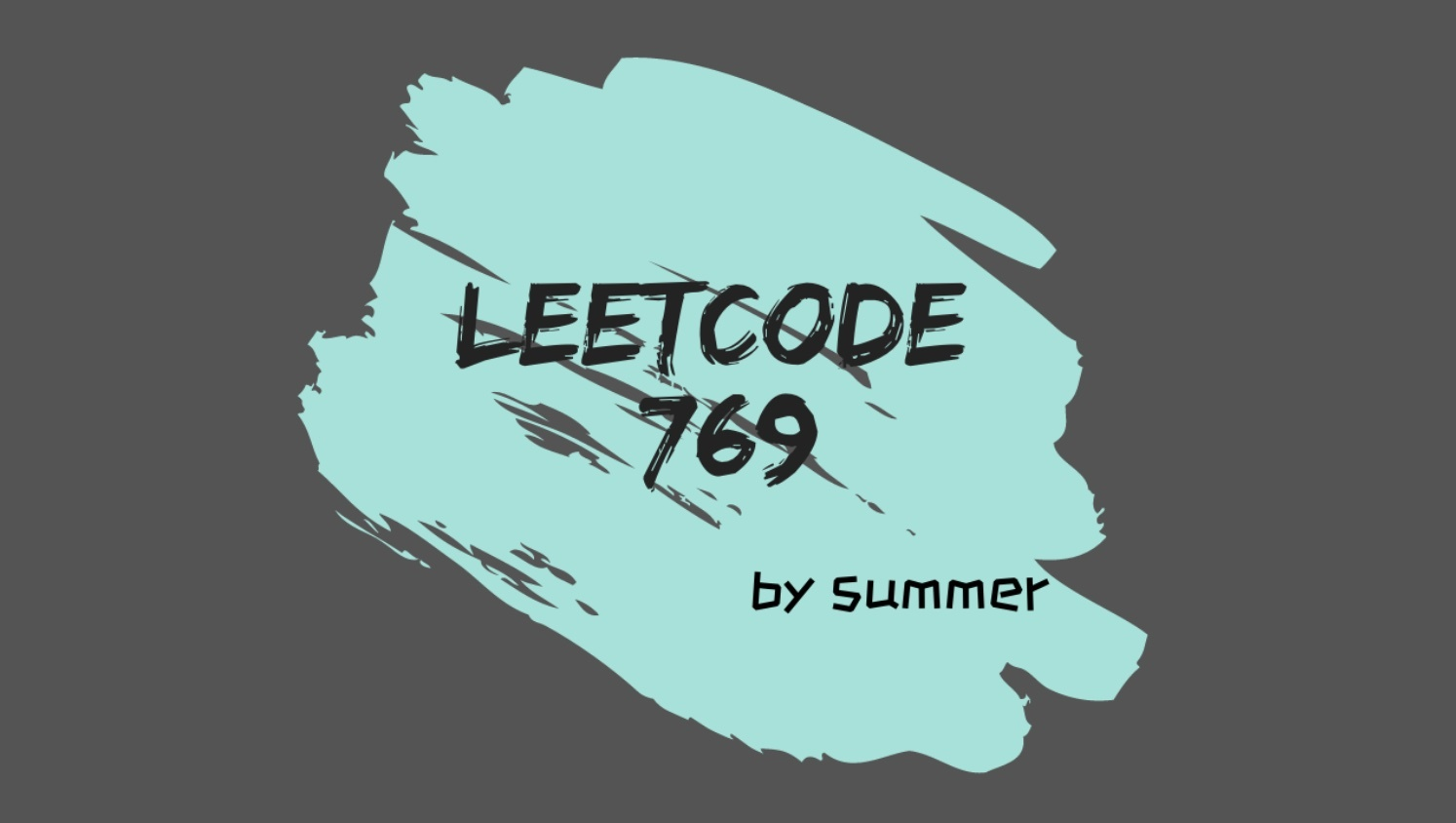# LeetCode 769. Max Chunks To Make Sortedliu_liu## 问题描述

输入：arr = [4,3,2,1,0]输出：1解释：最多只能划分为 1 个，因为分成多个区块后，连接后无法有序。

输入：arr = [1,0,2,3,4]输出：4解释：可将其划分为 [1, 0], , ,  4 个区块。

• arr 的长度为 [1, 10]

• arr[i][0, 1, ..., arr.lenth - 1] 的任意排列。

## 解题思路

### 解法 1

#### 思路分析

##### 单区块

• 对于 0 来说，0 < 2，肯定是需要划分到一个区块中，即 [2, 1, 0]。因为 0 单独成块后，无法有序。

• 对于 3 来说，3 > 2，可以单独成为一个区块。

##### 多区块

• 如果比某个区块最大值小，则需合并到该区块中，其后的区块也连同合并。因为区块是按顺序划分的。

• 如果比所有区块最大值都大，则可单独成块。

##### 思路总结

• 使用数组记录每个区块的最大值。

• 逐个对数字进行处理，合并或者添加区块，并更新区块最大值。

• 数组的长度即为最终区块个数。

#### 代码实现

js 代码实现如下：

var maxChunksToSorted = function (arr) {  if (arr && arr.length > 0) {    let maxNum = arr;    // 存储每一段的最大值    let chunksMaxNum = [arr];    var i = 1;    while (i <= arr.length - 1) {      let flag = false;      // 逐个与 chunksMaxNum 进行比较      let j = 0;      while (j < chunksMaxNum.length) {        if (arr[i] < chunksMaxNum[j]) {          // 标记为已处理          flag = true;          // 从 j 起，需要合并          // 最后一个最大值不删          chunksMaxNum.splice(j, chunksMaxNum.length - j - 1);          break;        }        j += 1;      }      // 可单独为 chunk      if (!flag) {        chunksMaxNum.push(arr[i]);      }      i += 1;    }    return chunksMaxNum.length;  }  return 0;};

### 解法 2

#### 思路分析

最大值： [1, 3, 3, 3, 4]排序后： [0, 1, 2, 3, 4]

#### 代码实现

java 实现代码如下：

public class Solution {    public int maxChunksToSorted(int[] arr) {        if (arr != null && arr.length > 0) {            // 保存当前位置最大值            int[] maxNum = new int[arr.length];            int max = Integer.MIN_VALUE;            int result = 0;            for (int i = 0; i < arr.length; i++) {                // 计算最大值                max = Math.max(max, arr[i]);                maxNum[i] = max;            }            for (int i = 0; i < maxNum.length; i++) {                // 如果刚好等于排序后的数字，则可以单独成块                if (maxNum[i] == i) {                    result += 1;                }            }            return result;        }        return 0;    }}## 评论